# Calculate Short Circuit Current of any Transformer in just 3 steps

Calculate Short Circuit Current of any Transformer in just 3 steps 1024 576 Gaurav J

In this tutorial I’ll explain three simple steps to calculate short circuit current of any transformer. It will also help you in deciding circuit breaker rating. So, let’s start !

## Step 1

##### Get the following details
• KVA/MVA rating of a transformer (for understanding purpose assume it is 100KVA)
• Secondary Voltage (Assue 440 volts)
• % Impedance (You’ll get it from the name plate of transformer, for our example assume 5%)

## Step 2

For 3 phase transformer, use following formula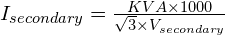For 1 phase transformer, use following formula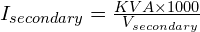Let’s calculate full load current in our example.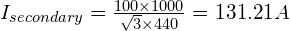## Step 3

##### Calculate the short circuit current

We’ll now calculate the actual short circuit current using the following formula.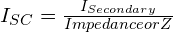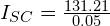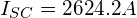So, this is our Short circuit current. This will help you to decide the rating of circuit breaker. In this case you need a breaker who’s fault current breaking capacity is more than 2624.1A or 2.6kA.

You can also calculate primary current of any transformer in just 2 steps, to know more about it, click here.###### Gaurav J

Electrical Engineer. Content Creator. Currently working with a High & Extra High Voltage Switchgear Industry.

All stories by: Gaurav J